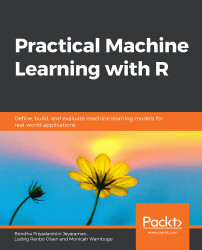•#### Practical Machine Learning with R#### Overview of this book

With huge amounts of data being generated every moment, businesses need applications that apply complex mathematical calculations to data repeatedly and at speed. With machine learning techniques and R, you can easily develop these kinds of applications in an efficient way. Practical Machine Learning with R begins by helping you grasp the basics of machine learning methods, while also highlighting how and why they work. You will understand how to get these algorithms to work in practice, rather than focusing on mathematical derivations. As you progress from one chapter to another, you will gain hands-on experience of building a machine learning solution in R. Next, using R packages such as rpart, random forest, and multiple imputation by chained equations (MICE), you will learn to implement algorithms including neural net classifier, decision trees, and linear and non-linear regression. As you progress through the book, you’ll delve into various machine learning techniques for both supervised and unsupervised learning approaches. In addition to this, you’ll gain insights into partitioning the datasets and mechanisms to evaluate the results from each model and be able to compare them. By the end of this book, you will have gained expertise in solving your business problems, starting by forming a good problem statement, selecting the most appropriate model to solve your problem, and then ensuring that you do not overtrain it.Free Chapter
An Introduction to Machine LearningData Cleaning and Pre-processingFeature EngineeringIntroduction to neuralnet and Evaluation MethodsLinear and Logistic Regression ModelsUnsupervised LearningAppendix## Summary

In this chapter, we learned how to perform several operations on a data frame, including scaling, standardizing, and normalizing. Also, we covered the sorting, ranking, and joining operations with their implementations in R. We discussed the need for pre-processing of the data; and identified and handled outliers, missing values, and duplicate values.

Next, we moved on to the sampling of data. It is important for the data to contain a reasonable sample of each class that is to be predicted. If the data is imbalanced, it can affect our predictions in a negative manner. Therefore, we can use either the undersampling, oversampling, ROSE, or SMOTE techniques imbalanced to ensure that the dataset is representative of all the classes that we want to predict. This can be done using the MICE, rpart, ROSE, and caret packages.

In the next chapter, we will cover feature engineering in detail, where we will focus on extracting features to create models.